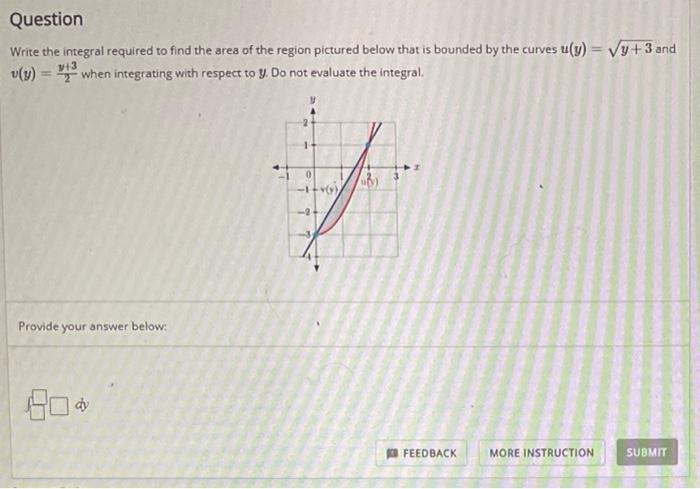# Question Solved1 AnswerQuestion Write the integral required to find the area of the region pictured below that is bounded by the curves u(y) = Vy+3 and v(y) = 3 when integrating with respect to y. Do not evaluate the integral. 13 Provide your answer below: Do. dy FEEDBACK MORE INSTRUCTION SUBMITTranscribed Image Text: Question Write the integral required to find the area of the region pictured below that is bounded by the curves u(y) = Vy+3 and v(y) = 3 when integrating with respect to y. Do not evaluate the integral. 13 Provide your answer below: Do. dy FEEDBACK MORE INSTRUCTION SUBMIT
More
Transcribed Image Text: Question Write the integral required to find the area of the region pictured below that is bounded by the curves u(y) = Vy+3 and v(y) = 3 when integrating with respect to y. Do not evaluate the integral. 13 Provide your answer below: Do. dy FEEDBACK MORE INSTRUCTION SUBMIT